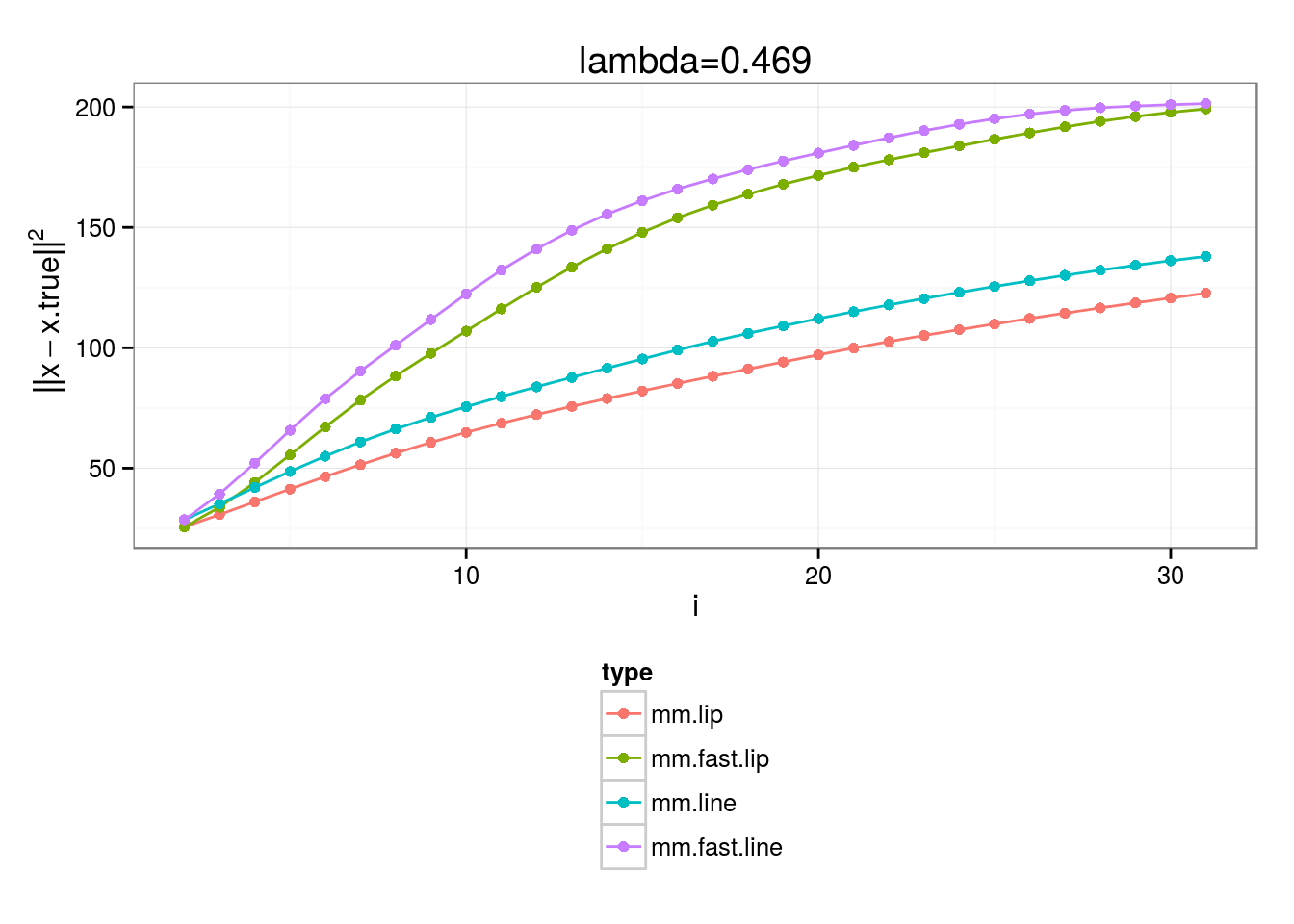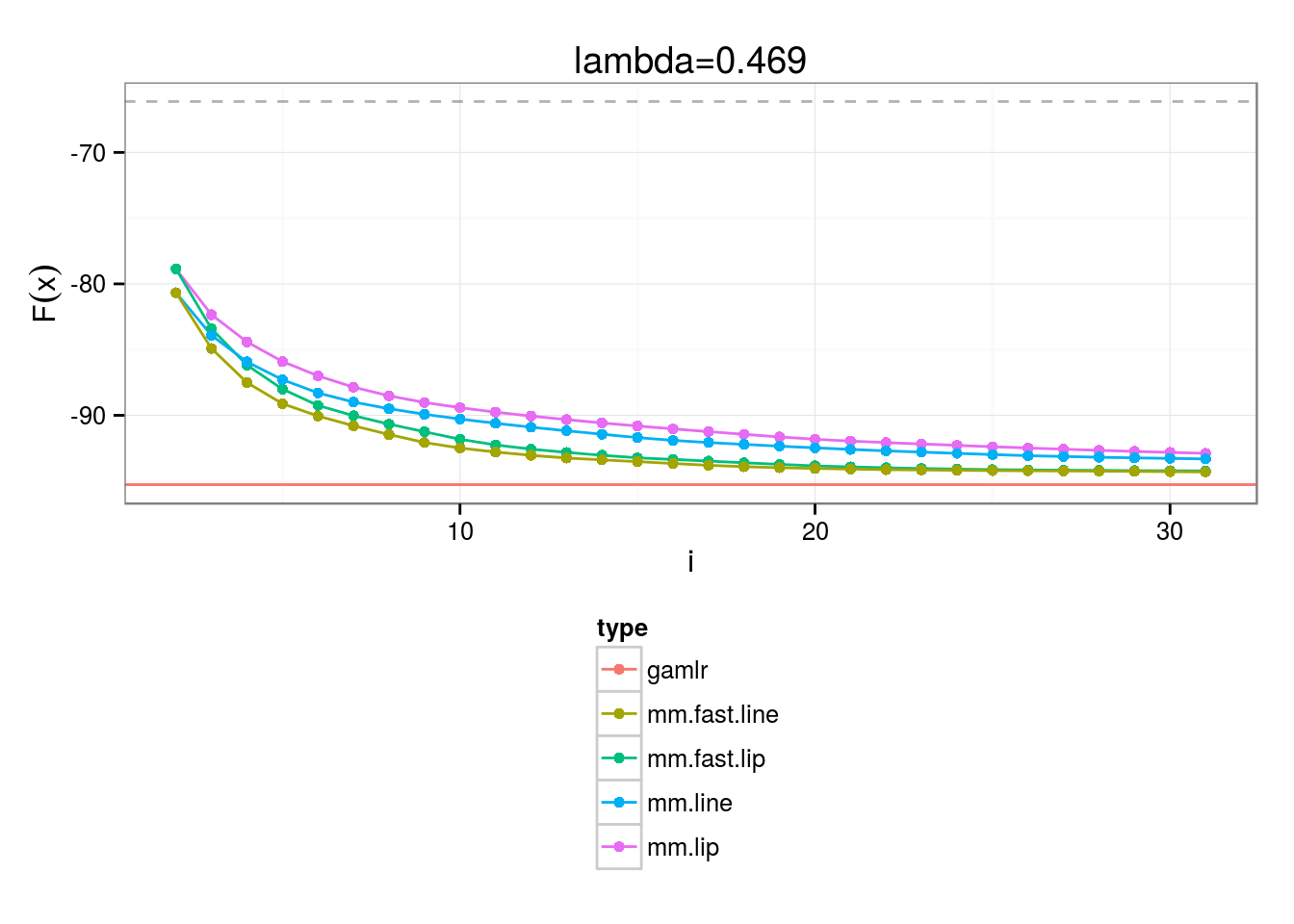$\newcommand{\argmin}{\operatorname{argmin}} \newcommand{\sign}{\operatorname{sign}} \newcommand{\diag}{\operatorname{diag}(#1)} \newcommand{\prox}{\operatorname{prox}_{#1}\left(#2\right)}$

# Introduction

We’re going to demonstrate some of the material from the BayesMAP paper 1 for the Logit model.

Let’s say we have $$M$$ observations, a matrix $$A$$ for $$N$$ predictors, $$y_i$$ successes, each with $$m_i$$ trials: $\begin{gather*} \argmin_x \left\{ F(x) = f(x) + \lambda \phi_q(x) \right\} \end{gather*}$ with $$\phi_q(x_j) = \log(1+|x_j|/q)$$ and \begin{align} f(x) &= \sum_{i=1}^N m_i \log\left(1+e^{a^T_i x}\right) - y_i a_i^T x \notag\\ &= \sum_{i=1}^N m_i \log\left(\cosh(a^T_i x/2)\right) - \kappa_i a_i^T x - \log(2) \label{eq:log-lik} \end{align} and $$\kappa_i = y_i-m_i/2$$.

We use the Proximal Gradient algorithm (described here) to estimate the $$\argmin$$.

Note: $$\phi_q(x)$$ is not convex but is quasi-convex instead, and convergence may be provable.

The $$\operatorname{prox}$$ operator, in this case the double-Pareto penalty, has an exact solution given component-wise by \begin{align*} \prox{\lambda \phi_q}{u} &= \frac{\sign(u)}{2} \left(|u|-q+\sqrt{(q-|u|)^2 + 4\max(q |u|-\lambda, 0)}\right) \end{align*} In order to actually use the Proximal Gradient algorithm, we need to specify a surrogate quadratic-form $$Q(x,z)$$ for $$f(x)$$ (detailed here), and, thus, its square and linear terms $$\Lambda(z)$$ and $$\eta(z)$$, respectively.

Inspired by the half-quadratic, i.e. Geman-Yang (GY) and Geman-Reynolds (GR), Variational Bayes and Majorization literature, which use a combination of techniques to obtain envelopes that are quadratic in the “dual” argument, we consider the following $$Q(x,y)$$:

### Lipschitz/MM

For this model, $$f(x)$$, the negative log likelihood in $$\eqref{eq:log-lik}$$, has Lipschitz continuous gradient (see here for more information) with $$L \ge L_f = \sigma(A^T A)/4$$, where $$\sigma(B)$$ is the largest eigenvalue of $$B$$, and quadratic-form terms $$\Lambda(z) = A^T A \cdot L$$, $$\eta(z)^T = \left(m \operatorname{logit}^{-1}(Az) - y\right)^T A$$

### GY

The GY-inspired approach: \begin{align*} \log\left(\cosh(x/2)\right) = \inf_{z \ge 0} \left\{ \frac{1}{2}(x-z)^2 - \theta(z) \right\} \end{align*} which has the same quadtratic-form terms as Lipschitz/MM. However, in this case, we solve the inverse problem given by $$\Lambda^{-1} \eta$$ on each step, i.e. $y^{(i+1)} = x^{(i)} - \frac{1}{4} \left(A^T A\right)^{-1} \eta(x^{(i)})$

### GR

\begin{align*} \log\left(\cosh(x/2)\right) = \inf_{z \ge 0} \left\{ \frac{z}{2} x^2 - \theta(z) \right\} \end{align*} where $$\hat{z} = \tanh(x/2)/2x$$. Better yet, this form implies a quadratic upper bound given by 2. \begin{align*} \log\left(1+e^{-x}\right) &\le \log(1+e^{-z})+g(z)(x^2-z^2)-\frac{1}{2}(x-z) \\ &= Q(x,z) \end{align*} for $$g(z) = \frac{1}{2z}\left(\frac{1}{1+e^{-z}} - \frac{1}{2}\right)$$. Using this bound we get the desired form with $$\Lambda(z) = \sum_{i=1}^N m_i g(a_i^T z)a_i a_i^T$$ and $$\eta(z)^T = \sum_{i=1}^N \left(y_i - \frac{m_i}{2}\right) a_i^T$$.

The basic functions for this estimation problem are implemented by the following:

## Loading required package: knitr
logit.pareto.obj.fun = function(x, env) {
return(logit.loss.fun(x,env) + env$lambda * sum(log(1+abs(x)/env$q)))
}

return(diag(env$A)*sum(-env$kappa + 0.5 * env$m * tanh(env$A %*% x))
- env$lambda/(env$q-x))
}

x.grad = t(env$A) %*% (env$m * plogis(env$A %*% x) - env$kappa)
}

mm.logit.xi = function(x, env) {
xi.res = crossprod(env$A, env$m * plogis(env$A %*% x) - env$y)
return(xi.res)
}

require(plyr)
gr.logit.xi = function(x, env) {
Ax = env$A %*% x aaT = mget("aaT", env=list2env(env), mode="any", list(function(x) { alply(env$A, 1, function(a) tcrossprod(a))
}),
inherits=TRUE)[]
eta = mget("eta", env=list2env(env), mode="any",
list(function(x) {
Reduce("+", Map(function(b,a) b*a, env$kappa, alply(env$A,1)))
}),
inherits=TRUE)[]
#
# we use the limit of g(z) as z -> 0, which is 1/8
#
mgAz = env$m * ifelse(abs(Ax) < 1e-5, 1/8, (plogis(Ax)-0.5)/(2*Ax)) maaT = Map("*", mgAz, aaT) Lambda = Reduce('+', maaT) xi.t = both.solve(Lambda, eta) return(xi.t) } double.pareto.prox = function(x, lambda, env) { d = pmax(env$q * abs(x) - lambda, 0)
return(sign(x)/2 * (abs(x) - env$q + sqrt((env$q - abs(x))^2 + 4*d)))
}

obj.fun = logit.pareto.obj.fun
obj.fun.grad = logit.pareto.obj.fun.grad

# Simulate a Signal

$$x^*$$ is the true signal, so that $$A x^* = y$$, and sparsity is induced by sampling 30 non-zero $$N(0,1)$$ terms.

Our observation matrix $$A$$ is 100x300, comprised of $$\operatorname{N}(0,1/300)$$ entries and observations are sampled using $$y \sim \operatorname{Binom}\left(m,\frac{1}{1+e^{A x^*}}\right)$$

We determine the Lipschitz bound $$L_f$$ to be 2.5863 and set $$\lambda=0.469$$ and the double-Pareto parameter $$q=1$$.

## Comparisons

We compute a baseline minimum objective value by solving the following subgradient problem:

opt.obj = optim(prob.env$x.true, fn=obj.fun, gr=obj.fun.grad, prob.env, method="BFGS", control=list('maxit'=5000, 'trace'=1)) The resulting objective value is -66.1258. The Gamma Lasso has a similar form and thus is a good candidate for comparision. We use the gamlr package in what follows. require(gamlr) ## Loading required package: gamlr ## Loading required package: Matrix gamlr.results = gamlr(prob.env$A, prob.env$y/prob.env$m, family="binomial", gamma=1,
nlambda=100, maxit=5000, lambda.start=prob.env$lambda) x.gamlr = gamlr.results$beta[,dim(gamlr.results\$beta)]
obj.fun.gamlr = obj.fun(x.gamlr, prob.env)

## Algorithms

First, we source the code for our generic algorithms, e.g. Nesterov acceleration, back-tracking line-search, etc., (see the algos page for more information):

## Plots1. “BayesMAP” Walter Dempsey, Nicholas Polson, Brandon Willard

2. “A variational approach to Bayesian logistic regression models and their extensions” Jaakkola and Jordan link